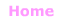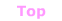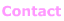Aa Bb Cc Dd Ee Ff Gg Hh Ii Jj Kk Ll Mm Nn Oo Pp Qq Rr Ss Tt Uu Vv Ww Xx Yy Zz

Ss
S.I.
sales tax
sample, random sample
scalars and vectors
sample space
scale, scale factor
scalene triangle
scatter diagram, scatter plot
scientific notation
score
seasons
secant, sec
second
secondary data set
section, cross-section
sector
sector graph
segment of a circle
segment of a line
semicircle
semiprime
senary
septagon
septenary
sequences
sequence rules
series
set
seven, seventeen, seventy
shape, shapes
shape of a distribution
sharing
side
side view
sieve of Eratosthenes
significant digits
signs and symbols
similar
similarity tests for triangles
similarity transformation
simple interest
simplify, simplest form
simultaneous equations
sine, sin
sine rule
six, sixteen, sixty
size
skewed data
skew lines
skip counting
slide
slope, slope-intercept form
smaller
solids, 3D shapes
solstice
solution, solve
solving equations
space
speed
sphere
spiral
split strategy
spring balance, spring scale
square
square measures

square number
square root
standard deviation
standard form
standard form of an equation
standard index form (UK)
standard notation
standard units of measurement
statistics
stem-and-leaf plot
step graph
straight angle
straight line
strategy
subitising
substitution
subtended angle
subtraction, subtrahend
subtraction by decomposition
subtraction properties
successive
sum
summary statistics
supplementary angles
surd
surface
surface area
survey
symbols and signs
symmetry
system of equations© Jenny Eather 2014. All rights reserved.